Tutorial math and reading software for elementary and secondary arithmetic, basic math, algebra, geometry, precalculus plus GED, ABE, and CLEP preparation for elementary school, high school, college, adult education, and homeschool students.

# Calculus Basics - Calculus Help - Pre Calculus Review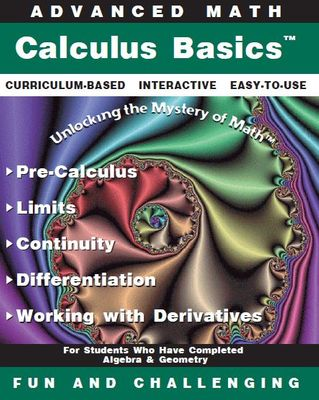Item# CalculusBasics
Regular price: \$59.00
Limited Time Offer: \$29.50
Media type::

## Product Description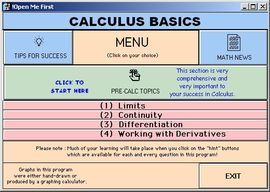Learning Calculus Basics with interactive calculus help is the seventh program in the Advanced Math Series. Pre Calculus is reviewed to prepare the student for the rigors of calculus. The pre calculus review contains instruction, sample problems and solutions, interactive practice problems, complete step by step solutions. The pre calculus topics with interactive pre calculus help:
-- Inequalities (solving, combined, absolute value, graphing solutions, polynomial inequalities)
-- Exponents (negative powers, fractional powers, irrational exponents)
-- Functions
-- Distance and Midpoint
-- Factoring and Solving
-- Complex Algebraic Fractions

Once the student has completed the pre calculus review, the student will begin the study of calculus with the foundation necessary for success. The calculus basics sections with step by step calculus help are:
-- Limits
-- Continuity
-- Differentiation:
---- Calculus Derivatives & The Slope
---- Differentiation Rules
---- Product Rule
---- Quotient Rule
---- The Chain Rule
---- Higher Order Calculus Derivatives
-- Working with Derivatives

Explanations are followed by caculus basics practice problems in which students are offered step by step calculus help. All pre-calculus and calculus problems are solved - never leave a question without knowing the solution and how to get there with no steps left out.
Available for immediate download or CD or online.## Accessories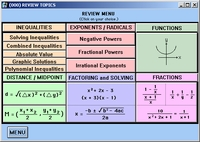Comprehensive Pre-Calc Review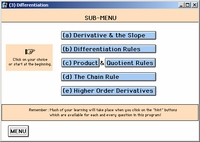Differentiation Sub-Menu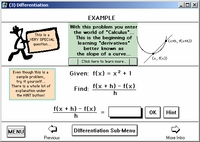Examples with Student Interaction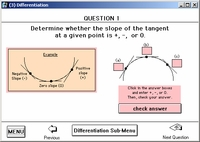Clear questions with immediate responses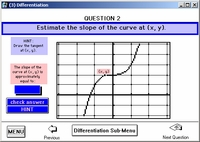Questions are varied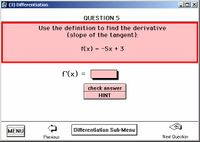HINTS are readily available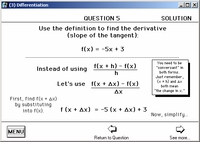Solutions unfold step by step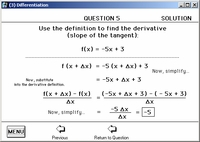All steps of the solutions are revealedat the student's request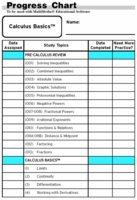Progress Charts to track work